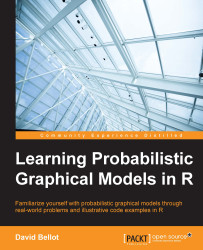•#### Learning Probabilistic Graphical Models in R#### Overview of this book

Probabilistic graphical models (PGM, also known as graphical models) are a marriage between probability theory and graph theory. Generally, PGMs use a graph-based representation. Two branches of graphical representations of distributions are commonly used, namely Bayesian networks and Markov networks. R has many packages to implement graphical models. We’ll start by showing you how to transform a classical statistical model into a modern PGM and then look at how to do exact inference in graphical models. Proceeding, we’ll introduce you to many modern R packages that will help you to perform inference on the models. We will then run a Bayesian linear regression and you’ll see the advantage of going probabilistic when you want to do prediction. Next, you’ll master using R packages and implementing its techniques. Finally, you’ll be presented with machine learning applications that have a direct impact in many fields. Here, we’ll cover clustering and the discovery of hidden information in big data, as well as two important methods, PCA and ICA, to reduce the size of big problems.
Learning Probabilistic Graphical Models in RCreditswww.PacktPub.comPrefaceFree Chapter
Probabilistic ReasoningExact InferenceLearning ParametersBayesian Modeling – Basic ModelsApproximate InferenceBayesian Modeling – Linear ModelsProbabilistic Mixture ModelsAppendixIndex## Beta-Binomial

The Beta-Binomial prior is another example and a well-known model where we set a prior distribution on the parameter of the distribution of interest. Here we are going to use a binomial distribution with a θ parameter. The θ parameter can be seen as a probability that an event will occur or not, or a proportion of the positive events in a sequence of experiments. Therefore, the parameter θ takes values in [0,1].

Let's first review the Binomial distribution with a simple example: let's say we have a coin and we want to know if the coin is impartial or not when we play the heads or tails game. The game is to toss the coin N times and try to estimate what is the probability θ of obtaining a head. This problem is very important because it is the basis of many other models. You can replace the game of heads or tails with the result of experimentation (positive or negative), the result of a poll (yes or no), or any other binary answer.

Historically, this model has been studied by Thomas...